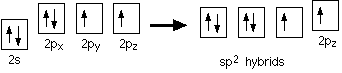# What is the hybridization in "CO"_2?

Jul 2, 2014

The carbon atom has $s p$ hybridization; the $\text{O}$ atoms have $s {p}^{2}$ hybridization.

#### Explanation:

You must first draw the Lewis structure for ${\text{CO}}_{2}$.According to VSEPR theory, we can use the steric number $\left(\text{SN}\right)$ to determine the hybridization of an atom.

$\text{SN}$ = number of lone pairs + number of atoms directly attached to the atom.

• $\text{SN = 2}$ corresponds to $s p$ hybridization.
• $\text{SN"= 3}$ corresponds to $s {p}^{2}$ hybridization.

We see that the $\text{C}$ atom has $\text{SN = 2}$. It has no lone pairs, but it is attached to two other atoms.

It has $s p$hybridization.

Each $\text{O}$ atom has $\text{SN = 3}$. It has 2 lone pairs and is attached to 1 $\text{C}$ atom.

Just as the carbon atom hybridized to form the best bonds, so do the oxygen atoms.

The valence electron configuration of $\text{O}$ is $\left[\text{He}\right] 2 {s}^{2} 2 {p}^{4}$.

To accommodate the two lone pairs and the bonding pair, it will also form three equivalent $s {p}^{2}$ hybrid orbitals.Two of the $s {p}^{2}$ orbitals contain lone pairs, while the remaining $s {p}^{2}$ orbital and the unhybridized $p$ orbital have one electron each.

We can see this arrangement in the $\text{C=O}$ bond of formaldehyde, which is equivalent to the right-hand side of the $\text{O=C=O}$ molecule.(from www.slideshare.net)

There is a similar arrangement on the left side of the $\text{O=C=O}$ molecule, but the $\pi$ bond is horizontal rather than vertical.Here is a video about the hybridization of carbon dioxide.